cancel
Showing results for
Did you mean:

Fabric is Generally Available. Browse Fabric Presentations. Work towards your Fabric certification with the Cloud Skills Challenge.Frequent Visitor

## Row by row Conditional Formatting on a Matrix

Dear all,

I have a matrix formating issue.

I want to conditionally format my matirx row by row to show the monthly highest and smallest values for the years and months selected.

The result should look like this: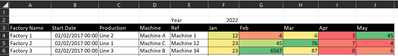I can get the correct formating (below) for the individual dates but not for the Month, by using this DAX expression.

_data rank =
IF (
NOT (ISBLANK ([Sum Ouput])),
RANKX(     CALCULATETABLE(
SUMMARIZE(
Table,
Table[Factory Name],
Table[Start Date],
Table[Production],
Table[Machine],
Table[Ref],
'Date'[Date]
),
"Summerised Data", [Sum Output]
),
ALLSELECTED('Date'[Date])
),
[Sum Output], , , Dense
)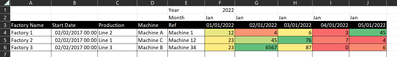Please can you help me rank the data so that I can see the ranking what the data is aggregated on a monthly basis rather than the granular daily values?

tagging a few people to help. @tamerj1@FreemanZ , @amitchandak

12 REPLIES 12Frequent Visitor

Hi,

Thank you for your reply and apologies for my delay in responding.

Please find a pbix file here with a sample data set: pbix file

Here is also an Excel file showing the row by row conditional formating that I want.

Ideally each row of data is conditionally formated based on the highest and lowest value in that row. This formatting should be maintained when I drill down to the day granualrity or drill up to month.Super User

For each Machine and Year you want to find the highest and lowest monthly values? Or for each Machine you want to find the highest and lowest values regardless of the year? You would need to use ISINSCOPE() to figure out where in the date hierarchy you are.Frequent Visitor

For each machine and year, I want to format the matrix so that the value in each row is formated according to how big it is from smallest to largests, ignoring other rows of data. as in the image below: For example for machine 2, 2023 46,466 data is the smallest and 2021 385,213 is the biggest.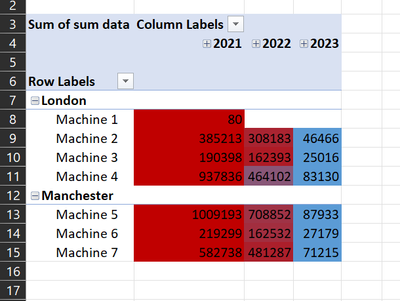I also what this for each month in a year - the value in each row should be formated from smallest to largest, ignoring other rows of data. Please see below example, for Machine two we get more detail than in the before image, we see that for 2022, June had the smallest data and March the largest.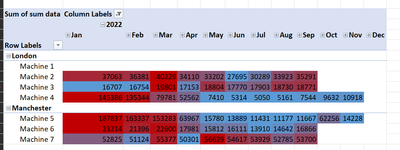If I drill down then the values in each row again should be formated to show the smallest to biggest, ignoring other rows of data. In the below eaxmple, the daily granulariy show that for Machine 2, in March 2022, March 20 had the smallest data whilst March 14 had the largest data.Super User

See attached.

color =
SWITCH(
TRUE(),
ISINSCOPE( 'Date Table'[Day Number] ),
VAR a =
CALCULATETABLE(
SUMMARIZE(
'Date Table',
'Date Table'[Year],
'Date Table'[Month],
'Date Table'[Day Number],
"sd", [sum data]
),
REMOVEFILTERS(
'Date Table'[Year],
'Date Table'[Month],
'Date Table'[Day Number]
)
)
RETURN
DIVIDE( [sum data] - MINXa, [sd] ), MAXXa, [sd] ) - MINXa, [sd] ),
ISINSCOPE( 'Date Table'[Month] ),
VAR a =
CALCULATETABLE(
SUMMARIZE(
'Date Table',
'Date Table'[Year],
'Date Table'[Month],
"sd", [sum data]
),
REMOVEFILTERS( 'Date Table'[Year], 'Date Table'[Month] )
)
RETURN
DIVIDE( [sum data] - MINXa, [sd] ), MAXXa, [sd] ) - MINXa, [sd] ),
ISINSCOPE( 'Date Table'[Year] ),
VAR a =
CALCULATETABLE(
SUMMARIZE( 'Date Table', 'Date Table'[Year], "sd", [sum data] ),
REMOVEFILTERS( 'Date Table'[Year] )
)
RETURN
DIVIDE( [sum data] - MINXa, [sd] ), MAXXa, [sd] ) - MINXa, [sd] )
)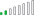Resolver II
Hi @lbendlin ! The code doesn't run properly when you select more than one year in a slicer. The reason is "color" measure isn't between 0 and 1. How could it be fixed?

Some days ago I created a similar question here:

Thank you so much for your time!Super User

I refactored the code. Seems to work better now.

``````color =
SWITCH (
TRUE (),
ISINSCOPE ( 'Date Table'[Day Number] ),
VAR a =
CALCULATETABLE (
SUMMARIZECOLUMNS ( 'Date Table'[Day Number] ),
REMOVEFILTERS ( 'Date Table'[Day Number] )
),
"sd", [sum data]
)
RETURN
DIVIDE ( [sum data] - MINX ( a, [sd] ), MAXX ( a, [sd] ) - MINX ( a, [sd] ) ),
ISINSCOPE ( 'Date Table'[Month] ),
VAR a =
CALCULATETABLE (
SUMMARIZECOLUMNS ( 'Date Table'[Month], 'Date Table'[Month Number] ),
REMOVEFILTERS ( 'Date Table'[Month], 'Date Table'[Month Number] )
),
"sd", [sum data]
)
RETURN
DIVIDE ( [sum data] - MINX ( a, [sd] ), MAXX ( a, [sd] ) - MINX ( a, [sd] ) ),
ISINSCOPE ( 'Date Table'[Year] ),
VAR a =
CALCULATETABLE (
SUMMARIZECOLUMNS ( 'Date Table'[Year] ),
REMOVEFILTERS ( 'Date Table'[Year] )
),
"sd", [sum data]
)
RETURN
DIVIDE ( [sum data] - MINX ( a, [sd] ), MAXX ( a, [sd] ) - MINX ( a, [sd] ) )
)
``````Resolver II

Hi @lbendlin! I just realized if I sort year column by other one, the code doesn't work properly.
I want to sort years inversely as you can see in the next image: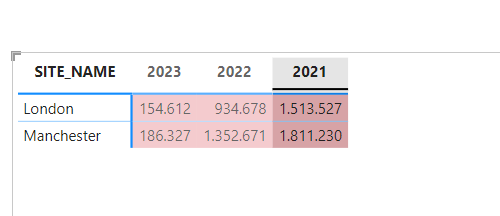How can I solve this? I doesn't understand why doesn't run. Thank you so much.Super User

You need to include the sort column in the formula, like you do for the month.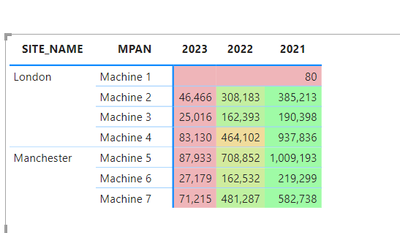Resolver II

thank you so much!!!😁Resolver II

That's perfect! Thank you so much for your time.Frequent Visitor

Hi Ibendlin,

Thank you so much for the time spent coming up with the above DAX. I appreciate your efort and time. Unfortunately, it doesn't work quite as I expect. In each row the data colouring doesn't flow from smallest to largest.

I created a work around with the below DAX. I essentially created two of the below DAX, one for formating the monthly view and another for formating the daily view and then added buttons for the use to move between the two matrices rather than drilling up or down. The below DAX is the monthly formating.

month Row by row CF =

VAR SummaryTable =

CALCULATETABLE(

Summarize(

'Data Table name',

'Data Table name'[row granularity column],

'Date Table'[Year],

'Date Table'[Month]

),

" SummaryTable column name", [sum measure of interest]

),

ALLSELECTED('Date Table') // column to remove filter so restart every row

)

VAR MaxValue =

MAXX (

SummaryTable,

[SummaryTable column name]

)

VAR MinValue =

MINX (

SummaryTable,

[SummaryTable column name]

)

VAR range = MaxValue - MinValue

VAR hue =

Round(

DIVIDE (

[sum measure of interest] - MinValue,

range

)*60, 0

) // + 240 // - to change the colour hue

VAR colour = "hsla(" & hue & ", " & "100%" & ", " &  "70%" & ", " & 1 & ")"

Return

colour

This solution is from Bas, How to Power BI video titled Unleash the full Potential of Conditional Formating- row by row colour scale in a Matrix.Super User

Please provide sample data that covers your issue or question completely, in a usable format (not as a screenshot).
https://community.powerbi.com/t5/Community-Blog/How-to-provide-sample-data-in-the-Power-BI-Forum/ba-...
Please show the expected outcome based on the sample data you provided.Announcements#### Power BI Monthly Update - November 2023

Check out the November 2023 Power BI update to learn about new features.#### Fabric Community News unified experience

Read the latest Fabric Community announcements, including updates on Power BI, Synapse, Data Factory and Data Activator.#### The largest Power BI and Fabric virtual conference

130+ sessions, 130+ speakers, Product managers, MVPs, and experts. All about Power BI and Fabric. Attend online or watch the recordings.Top Solution Authors
Top Kudoed Authors
Users online (3,153)